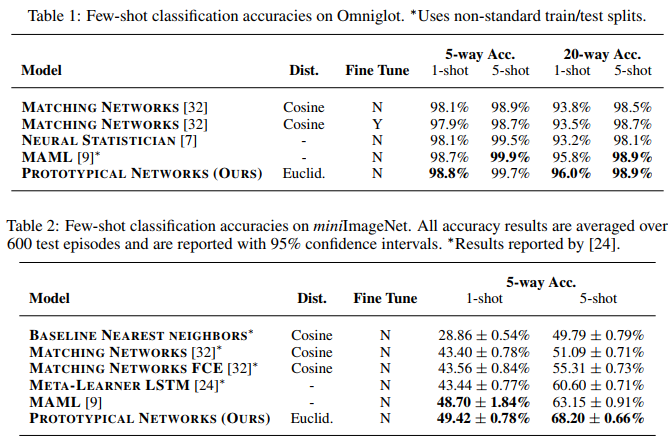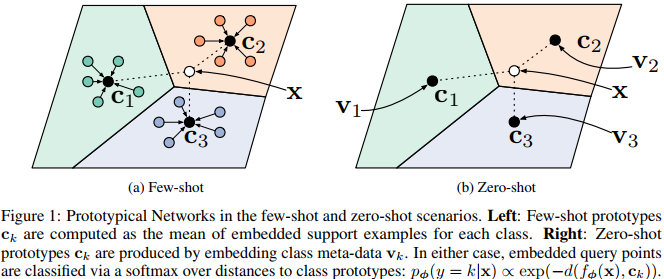## Summary

This paper proposes Prototypical Networks for the problem of few-shot (and zero-shot) classification, where a classifier must generalize to new data given only a small number of examples of each class. Prototypical Networks learn a metric space in which classification can be performed by computing distances to prototype representations of each class. The proposed method is relatively intuitive and surprisingly effective.

## Proposed method

In few-shot classification we are given a small support set of $$N$$ labeled examples $$S = {(x_1,y_1), ... ,(x_N, y_N)}$$ where each $$x_i \in R^D$$ is the D-dimensional feature vector of an example and $$y_i$$ is the corresponding label. $$S_k$$ denotes the set of examples labeled with class $$k$$.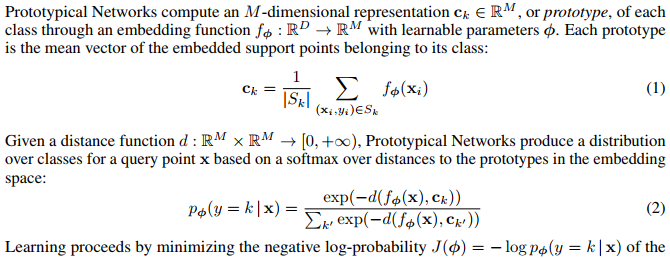Note that in their implementation, $$f_\phi$$ is a four-layer CNN.

Interestingly, when the system uses an Euclidean distance $$d(.)$$ the system becomes a linear model as illustrated in Figure 1.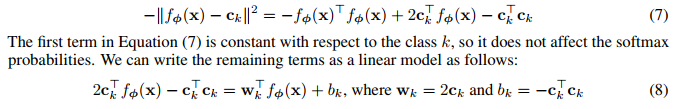The training algorithm is as follows: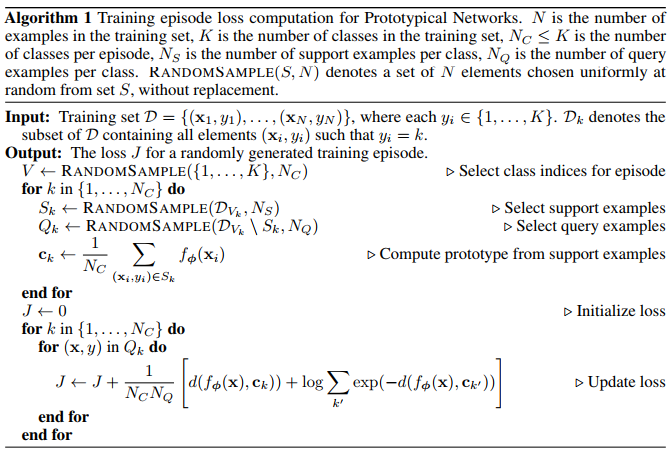A real illustration of the resulting embedding of a propotype network is shown in Fig.2.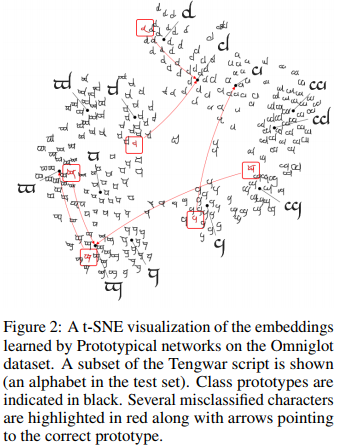## Results

The method was state of the art on several datasets back in 2017.